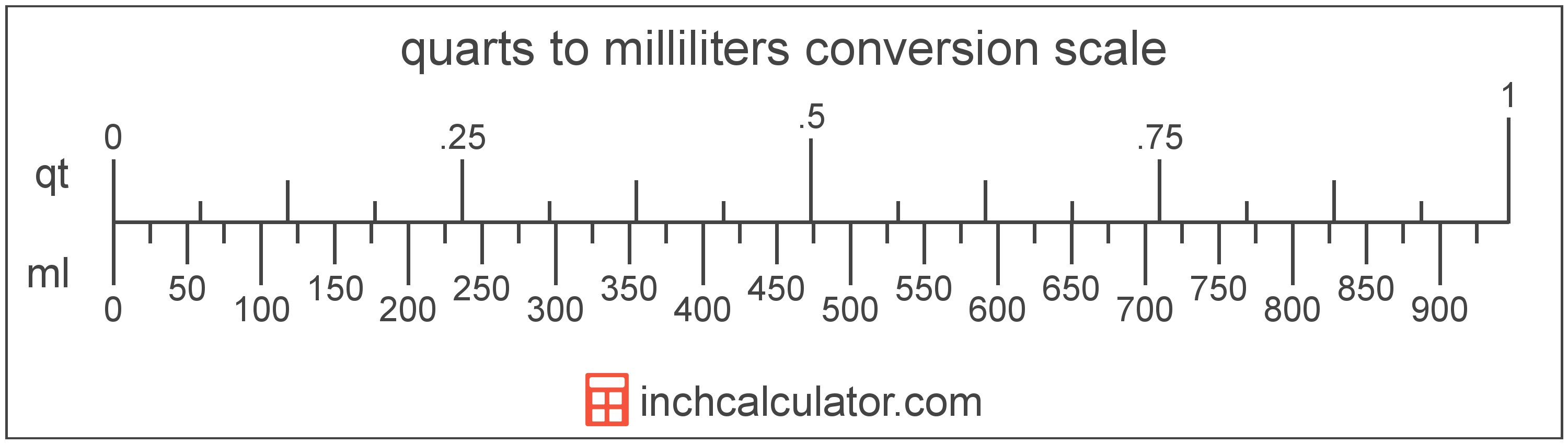# Milliliters to Quarts Converter

Enter the volume in milliliters below to get the value converted to quarts.

Results in Quarts:1 mL = 0.001057 qt

Do you want to convert quarts to milliliters?

## How to Convert Milliliters to Quarts

To convert a measurement in milliliters to a measurement in quarts, multiply the volume by the following conversion ratio: 0.001057 quarts/milliliter.

Since one milliliter is equal to 0.001057 quarts, you can use this simple formula to convert:

quarts = milliliters × 0.001057

The volume in quarts is equal to the volume in milliliters multiplied by 0.001057.

For example, here's how to convert 500 milliliters to quarts using the formula above.
quarts = (500 mL × 0.001057) = 0.528344 qt## What is a Milliliter?

The milliliter is a unit of volume equal to 1 cubic centimeter, 1/1,000 of a liter, or about 0.061 cubic inches.

The milliliter is an SI unit of volume in the metric system. In the metric system, "milli" is the prefix for thousandths, or 10-3. A milliliter is sometimes also referred to as a millilitre. Milliliters can be abbreviated as mL, and are also sometimes abbreviated as ml or mℓ. For example, 1 milliliter can be written as 1 mL, 1 ml, or 1 mℓ.

Since one milliliter is equivalent to one cubic centimeter, milliliters are sometimes expressed using the abbreviation for a cubic centimeter (cc) for things such as medical dosages or engine displacements.

## What is a Quart?

The US liquid quart is a unit of fluid volume equal to one fourth of a gallon, two pints, or four cups.

The liquid quart should not be confused with the dry quart (US) or the imperial quart, which are different units. However, when someone refers to a volume in quarts, they typically mean the US liquid quart.

The quart is a US customary unit of volume. Quarts can be abbreviated as qt; for example, 1 quart can be written as 1 qt.

## Milliliter to Quart Conversion Table

Table showing various milliliter measurements converted to quarts.
Milliliters Quarts
1 mL 0.001057 qt
2 mL 0.002113 qt
3 mL 0.00317 qt
4 mL 0.004227 qt
5 mL 0.005283 qt
6 mL 0.00634 qt
7 mL 0.007397 qt
8 mL 0.008454 qt
9 mL 0.00951 qt
10 mL 0.010567 qt
20 mL 0.021134 qt
30 mL 0.031701 qt
40 mL 0.042268 qt
50 mL 0.052834 qt
60 mL 0.063401 qt
70 mL 0.073968 qt
80 mL 0.084535 qt
90 mL 0.095102 qt
100 mL 0.105669 qt
200 mL 0.211338 qt
300 mL 0.317006 qt
400 mL 0.422675 qt
500 mL 0.528344 qt
600 mL 0.634013 qt
700 mL 0.739682 qt
800 mL 0.845351 qt
900 mL 0.951019 qt
1,000 mL 1.0567 qt

## References

1. National Institute of Standards and Technology, Specifications, Tolerances, and Other Technical Requirements for Weighing and Measuring Devices, Handbook 44 - 2019 Edition, https://nvlpubs.nist.gov/nistpubs/hb/2019/NIST.HB.44-2019.pdf